# Electric sail performance analysis

### This is a summary of our paper "Electric sail performance analysis" by G. Mengali, A. Quarta and P. Janhunen, Journal of Spacecraft and Rockets, in press, 2007.

Because of copyright restrictions, we cannot put the whole paper on the web. Here is thus only the abstract and the figures. Electronic PDF reprints can be asked from Pekka Janhunen, firstname.lastname@fmi.fi.

Abstract: An electric sail uses the solar wind dynamic pressure to produce a small, but continuous thrust by interacting with an electric field generated around a number of charged tethers (the electric sail). Due to the weakness of the solar wind dynamic pressure, quantifiable in about 2 nPa at Earth's distance from the Sun, the required tether length is \pekka{of} the order of some kilometers. Equipping a 100 kg spacecraft with one hundred of such tethers, each one being of 10 km length, is sufficient to obtain a spacecraft acceleration of about 1 mm/s^2. These values render the electric sail a potentially competitive propulsion means for future mission applications. The aim of this paper is to provide a preliminary analysis of the electric sail performance and to investigate the capabilities of this propulsion system in performing interplanetary missions. To this end the minimum time rendezvous transfer problem between circular and coplanar orbits is considered and the optimal steering law is found using an indirect approach. The main differences between electric sail and solar sail performance are also emphasized.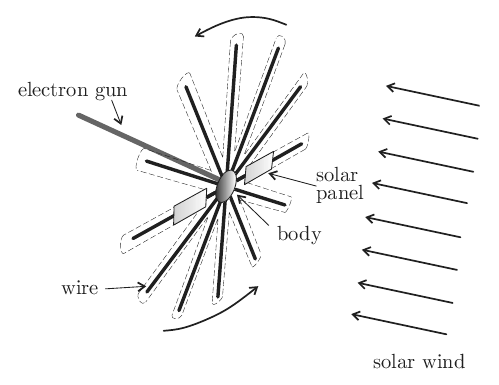Figure 1: Conceptual sketch of an electric sail.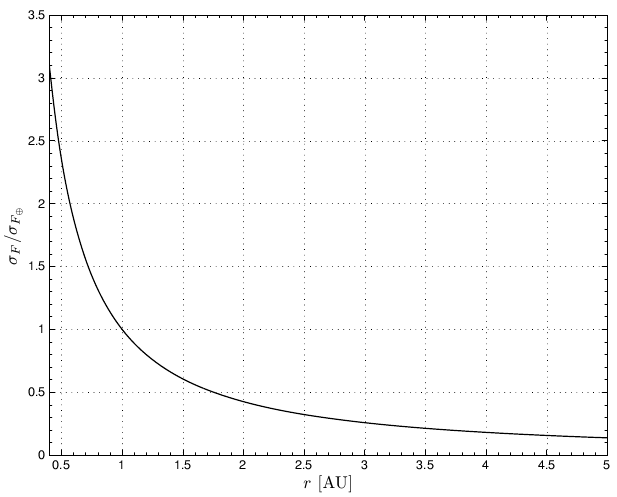Figure 2a: Propulsive force as function of Sun distance: Dimensionless propulsive force.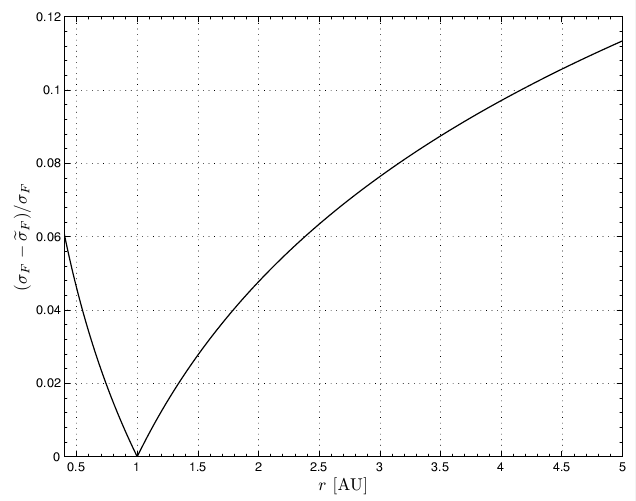Figure 2a: Accuracy of the simplified model, see Eq. (4).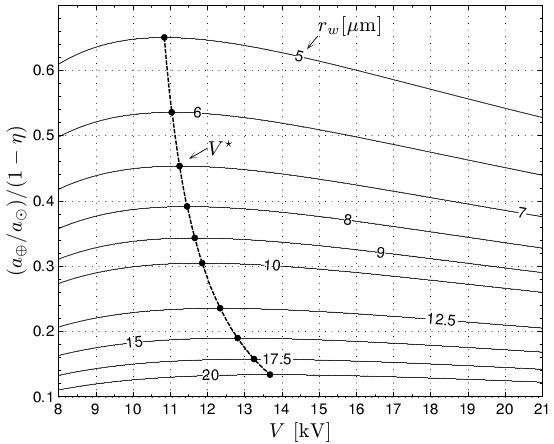Figure 3: Dimensionless propulsive acceleration at 1 AU (see Eq. (10)).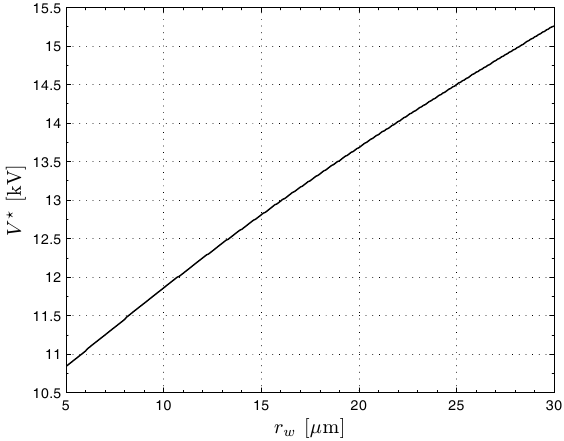Figure 4: Optimal tether voltage.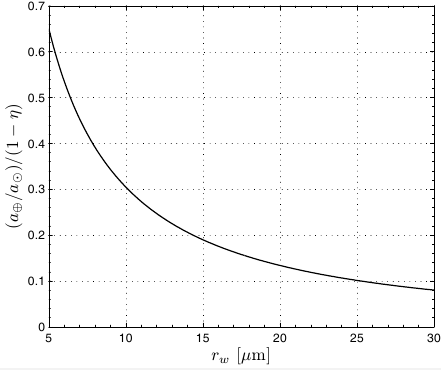Figure 5: Optimal dimensionless propulsive acceleration (see Eq. (12)).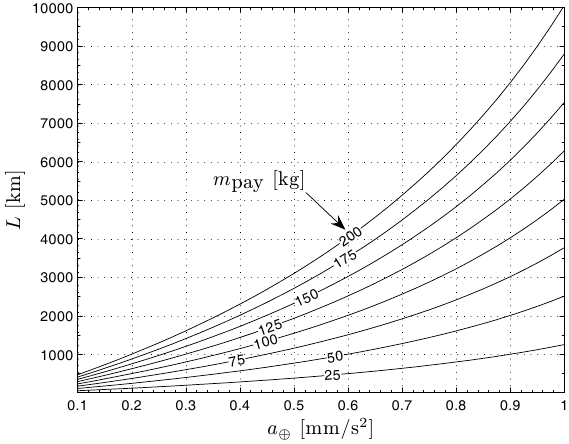Figure 6: Total tether length (rw=10 um).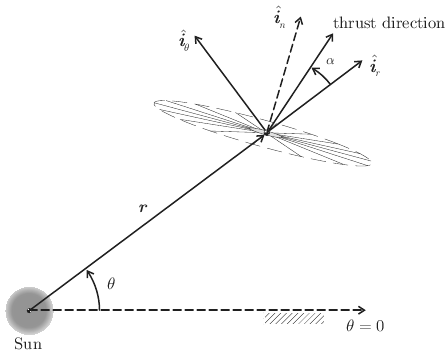Figure 7: Reference frame and thrust angle.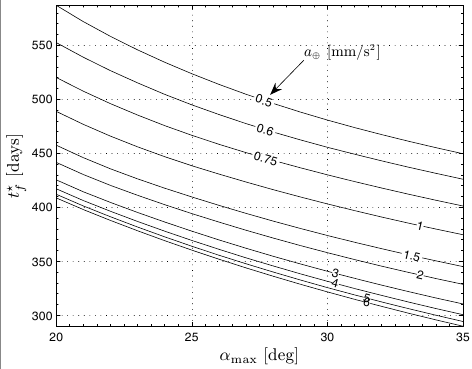Figure 8a: Minimum time circle-to-circle rendezvous: Earth-Mars transfer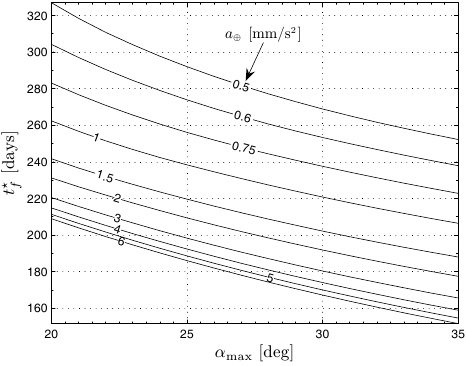Figure 8b: Minimum time circle-to-circle rendezvous: Earth-Venus transfer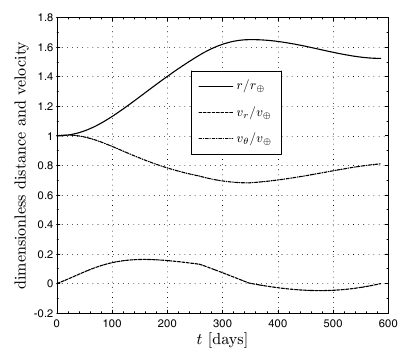Figure 9a: Earth-Mars circle-to-circle rendezvous with aEarth=0.5 mm/s^2 and alpha_max=20 deg: time histories of distance and velocity Figure 9b: Earth-Mars circle-to-circle rendezvous with aEarth=0.5 mm/s^2 and alpha_max=20 deg: acceleration components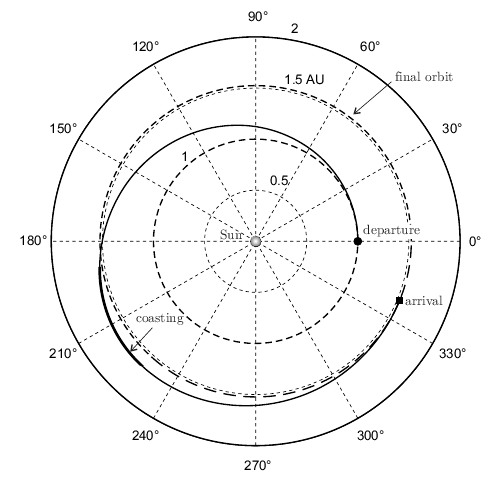Figure 10: Optimal trajectory for an Earth-Mars transfer using an electric sail.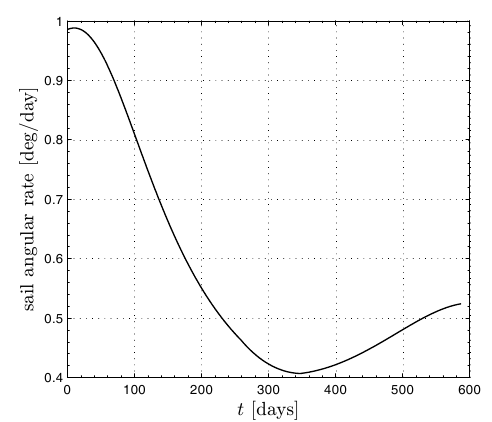Figure 11: Sail angular rate for an Earth-Mars circle-to-circle transfer.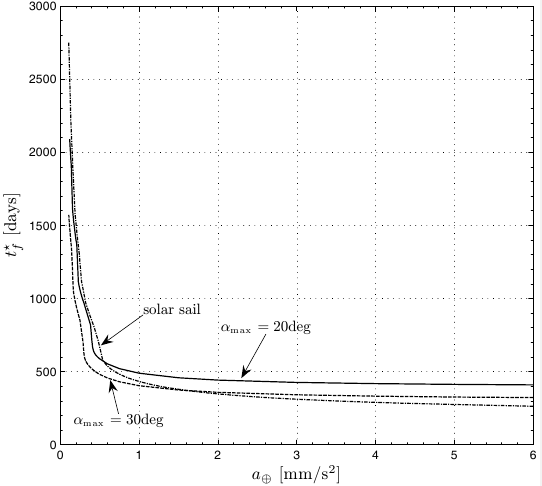Figure 12: Minimum time Earth-Mars transfer as a function of aEarth.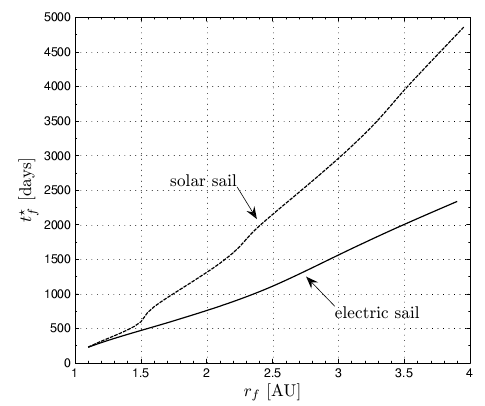Minimum transfer times for an electric sail (aEarth = 0.5 mm/s^2 and alpha_max=30 deg) and a solar sail.